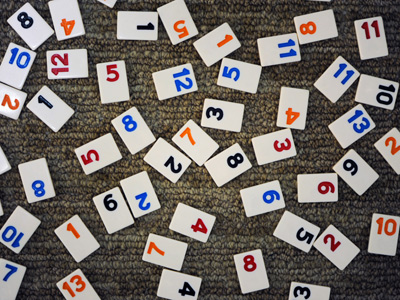987,642 is the largest number that can be made from the digits 2, 4, 6, 7, 8, 9.

# Compare and Order Numbers 2

This Math quiz is called 'Compare and Order Numbers 2' and it has been written by teachers to help you if you are studying the subject at elementary school. Playing educational quizzes is an enjoyable way to learn if you are in the 3rd, 4th or 5th grade - aged 8 to 11.

It costs only \$12.50 per month to play this quiz and over 3,500 others that help you with your school work. You can subscribe on the page at Join Us

Comparing and ordering numbers is useful when listing them by size. A good understanding of the value of a figure means you can compare one number with another and put several numbers in order. For example, a six in the hundreds column is worth 600 but in the thousands column it is worth 6,000.

1.
Which number is less than -34?
-32
-35
34
-1
With minus numbers the higher the figure the lower the number
2.
Which is the least amount?
54,697
54,796
54,976
54,967
All have 54 thousands but 54,697 has only 6 hundreds. The other three numbers have at least 7 hundreds
3.
What does the symbol ≤ mean?
Less than or equal to
More than or equal to
Equal to
Not equal to
The arrow points to the smaller number
4.
Which of these sequences is in ascending order?
56, 55, 54, 53, 52
455, 450, 445, 440, 435
0.1, 0.2, 0.3, 0.4, 0.5
23, 22, 21, 20, 19, 18
Ascending order means the numbers are getting larger
5.
Which number comes before -12 on a numberline?
-10
-11
-13
-9
-13 is one less than -12
6.
Which of these number comes first on a number line?
0.1
0.25
0.125
0.5
The smallest number will always come first on a number line
7.
Which of these sequences is in descending order?
5, 10, 15, 20, 25, 30
2.5, 2.6, 2.7, 2.8, 2.9
4,578, 4,579, 4,580, 4,581
68, 66, 64, 63, 62
Descending order means the numbers are getting smaller
8.
Which number is halfway between 27,400 and 28,000?
27,500
27,600
27,700
27,800
28,000 - 27,400 = 600
600 ÷ 2 = 300
27,400 + 300 = 27,700
9.
What is the largest number that can be made from the digits 2, 4, 6, 7, 8, 9?
986,742
968,742
978, 624
987,642
9 goes in the hundred thousands column
8 in the ten thousands column
7 in the thousands column
6 in the hundreds,
4 in the tens and
2 in the units
10.
What does the symbol ≥ mean?
Less than or equal to
More than or equal to
Equal to
Not equal to
The line beneath the more than symbol means the number could also be equal to
Author:  Amanda Swift